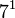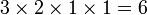# Groups of order 2520

## Contents

See pages on algebraic structures of order 2520| See pages on groups of a particular order

## Statistics at a glance

The number 2520 has the prime factorization:$\! 2520 = 2^3 \cdot 3^2 \cdot 5^1 \cdot 7^1 = 8 \cdot 9 \cdot 5 \cdot 7$

All groups of this order have not yet been classified. The information below is therefore partial.

Quantity Value Explanation
Total number of groups up to isomorphism unknown, but likely between 250 and 2500
Number of abelian groups (i.e., finite abelian groups) up to isomorphism 6 (Number of abelian groups of order$2^3$) times (Number of abelian groups of order$3^2$) times (Number of abelian groups of order$5^1$) times (Number of abelian groups of order$7^1$) = (number of unordered integer partitions of 3) times (number of unordered integer partitions of 2) times (number of unordered integer partitions of 1) times (number of unordered integer partitions of 1) =$3 \times 2 \times 1 \times 1= 6$. See classification of finite abelian groups and structure theorem for finitely generated abelian groups.
Number of nilpotent groups (i.e., finite nilpotent groups) up to isomorphism 10 (Number of groups of order 8) times (Number of groups of order 9) times (Number of groups of order 5) times (Number of groups of order 7) =$5 \times 2 \times 1 \times 1 = 10$. See number of nilpotent groups equals product of number of groups of order each maximal prime power divisor, which in turn follows from equivalence of definitions of finite nilpotent group.
Number of solvable groups (i.e., finite solvable groups) up to isomorphism unknown, but likely between 250 and 2500
Number of non-solvable groups up to isomorphism PLACEHOLDER FOR INFORMATION TO BE FILLED IN: [SHOW MORE] There are four possibilities for the simple non-abelian composition factor: alternating group:A7 (order 2520), alternating group:A6 (order 360), projective special linear group:PSL(3,2) (order 168), and alternating group:A5 (order 60). For each choice of simple non-abelian composition factor, the other composition factors must be cyclic of prime order so that the product is the order of the group.
Number of perfect groups up to isomorphism 1 alternating group:A7
Number of simple groups up to isomorphism 1 alternating group:A7
Number of almost simple groups up to isomorphism 1 alternating group:A7
Number of quasisimple groups up to isomorphism 1 alternating group:A7